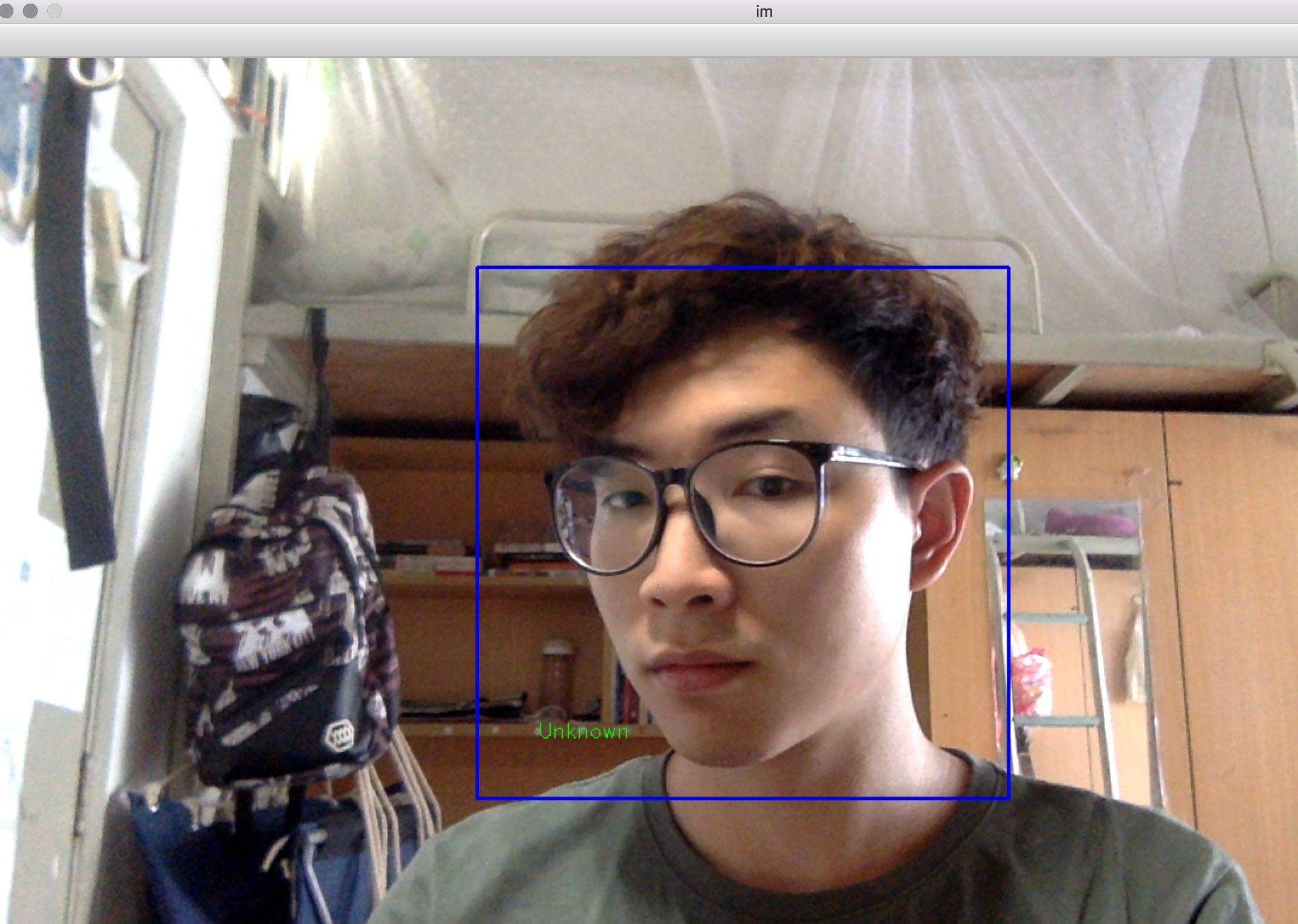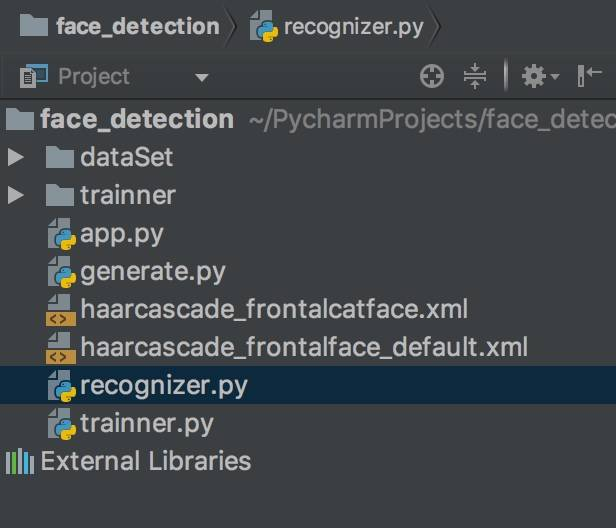# Python-OpenCV进行人脸识别

## 导入

``````import cv2
import numpy as np
``````

## 加载识别器

``````recognizer = cv2.face.LBPHFaceRecognizer_create()
# recognizer = cv2.createLBPHFaceRecognizer() # in OpenCV 2
# recognizer.load('trainner/trainner.yml') # in OpenCV 2
``````

``````cascade_path = "haarcascade_frontalface_default.xml"
``````

``````cam = cv2.VideoCapture(0)
``````

``````# font = cv2.cv.InitFont(cv2.cv.CV_FONT_HERSHEY_SIMPLEX, 1, 1, 0, 1, 1)
font = cv2.FONT_HERSHEY_SIMPLEX
``````

## 识别程序的主循环

• 从摄像头中获取图像
• 将图像转换为灰度图片
• 在图片中检测人脸
• 用识别器识别该人的id
• 将识别出人脸的id或名称用矩形在图片中标出来
``````while True:
gray = cv2.cvtColor(im, cv2.COLOR_BGR2GRAY)
for (x, y, w, h) in faces:
cv2.rectangle(im, (x - 50, y - 50), (x + w + 50, y + h + 50), (225, 0, 0), 2)
img_id, conf = recognizer.predict(gray[y:y + h, x:x + w])
# cv2.cv.PutText(cv2.cv.fromarray(im), str(Id), (x, y + h), font, 255)
cv2.putText(im, str(img_id), (x, y + h), font, 0.55, (0, 255, 0), 1)
cv2.imshow('im', im)
if cv2.waitKey(10) & 0xFF == ord('q'):
break
``````

`recognizer.predict`为预测函数，`putText`则是在图片上添加文字

## 更进一步

``````        img_id, conf = recognizer.predict(gray[y:y + h, x:x + w])
if conf > 50:
if img_id == 1:
img_id = 'jianyujianyu'
elif img_id == 2:
img_id = 'ghost'
else:
img_id = "Unknown"
# cv2.cv.PutText(cv2.cv.fromarray(im), str(Id), (x, y + h), font, 255)
cv2.putText(im, str(img_id), (x, y + h), font, 0.55, (0, 255, 0), 1)
``````

## 释放资源

``````cam.release()
cv2.destroyAllWindows()
``````

## 测试## 完整代码``````import cv2
import numpy as np

recognizer = cv2.face.LBPHFaceRecognizer_create()
# recognizer = cv2.createLBPHFaceRecognizer() # in OpenCV 2
# recognizer.load('trainner/trainner.yml') # in OpenCV 2

cam = cv2.VideoCapture(0)
# font = cv2.cv.InitFont(cv2.cv.CV_FONT_HERSHEY_SIMPLEX, 1, 1, 0, 1, 1) # in OpenCV 2
font = cv2.FONT_HERSHEY_SIMPLEX

while True:
gray = cv2.cvtColor(im, cv2.COLOR_BGR2GRAY)
for (x, y, w, h) in faces:
cv2.rectangle(im, (x - 50, y - 50), (x + w + 50, y + h + 50), (225, 0, 0), 2)
img_id, conf = recognizer.predict(gray[y:y + h, x:x + w])
if conf > 50:
if img_id == 1:
img_id = 'jianyujianyu'
elif img_id == 2:
img_id = 'ghost'
else:
img_id = "Unknown"
# cv2.cv.PutText(cv2.cv.fromarray(im), str(Id), (x, y + h), font, 255)
cv2.putText(im, str(img_id), (x, y + h), font, 0.55, (0, 255, 0), 1)
cv2.imshow('im', im)
if cv2.waitKey(10) & 0xFF == ord('q'):
break

cam.release()
cv2.destroyAllWindows()
``````#### 110011 · 02/16/2019 at 12:16#### striver · 03/17/2019 at 15:54#### fengziChen · 04/18/2019 at 15:39#### 煎鱼 · 04/18/2019 at 17:09

yml保存的是参数，我们把人脸看成是输入的自变量（x），id看成是输出的因变量（y），就像是解y=ax方程一样，已知x和y，求参数a。而实际上参数不只有一个a，有很多个，这样看来，录入的过程其实就是有多条方程式，且已知x和y，求出尽可能精确的a参数，然后存放在yml中。
识别的时候，就是从yml中提取a，输入图像自变量x，得到y，y就是id了，就是大概这样的一个过程。
往往你说的录入就是一个拟合的过程，就是参数a不能拿到精确的值，只能尽量精确。那么输入越多的照片来训练（录入），a的值就会越准确。